## A parachutist bails out and freely falls 63 m. Then the parachute opens, and thereafter she decelerates at 1.5 m/s2. She reaches the ground

Question

A parachutist bails out and freely falls 63 m. Then the parachute opens, and thereafter she decelerates at 1.5 m/s2. She reaches the ground with a speed of 3.3 m/s. (a) How long is the parachutist in the air

in progress 0
2 months 2021-07-29T10:35:40+00:00 1 Answers 4 views 0

(a) The parachutist spent 24.84 secs in air

(b) The height the fall begins is 472 m

Explanation:

Here is the complete question:

A parachutist bails out and freely falls 63 m. Then the parachute opens, and thereafter she decelerates at 1.5 m/s2. She reaches the ground with a speed of 3.3 m/s. (a) How long is the parachutist in the air  (b) At what height does the fall begin?

Explanation: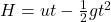Where H is the height

u is the initial velocity

t is the time

and g is the acceleration due to gravity (Take g = 9.8 m/s2)

Now, we can find the time spent before the parachute opens.

u = 0 m/s (we assume the parachutist starts from rest)

H = – 63 m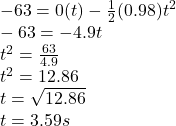This the time spent before the parachute opens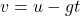where v is the final velocity

We can determine the final velocity before the parachute opens and she starts to decelerate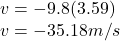Now, we will calculate the time spent after the parachute opens

From one of the equation of kinematics for linear motion,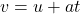Here, the initial velocity will be the final velocity just before the parachute opens, that is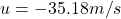From the question,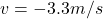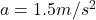We then get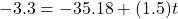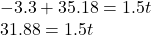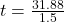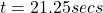(a) To determine how long the parachutist is in the air,

That is sum of the time used when falling freely and the time used after the parachute opens

= 3.59 secs + 21.25 secs

24.84 secs

Hence, the parachutist spent 24.84 secs in air

(b) To determine what height the fall begins

First, we will calculate the height from which the parachute opens

From one of the equation of kinematics for linear motion,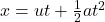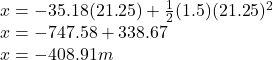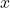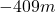Hence, the height the fall begins is 63m + 409m

= 472 m